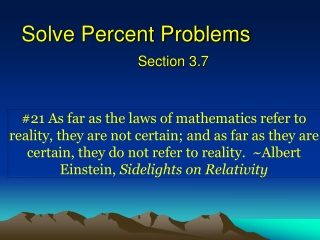Download PresentationSolve Percent Problems

# Solve Percent Problems

Download Presentation## Solve Percent Problems

- - - - - - - - - - - - - - - - - - - - - - - - - - - E N D - - - - - - - - - - - - - - - - - - - - - - - - - - -
##### Presentation Transcript

1. Solve Percent Problems Section 3.7

2. Vocabulary • Ratio • Uses division to compare two quantities. These ratios can be expressed in several different ways. They include: • Subtraction Property • Subtracting the same number to each side of an equation produces an equivalent equation • If x+a=b, then x+a-a=b-a, or x=b-a

3. Vocabulary • Percentages • A ratio or fraction whose denominator is 100 • Subtraction Property • Subtracting the same number to each side of an equation produces an equivalent equation • If x+a=b, then x+a-a=b-a, or x=b-a

4. Vocabulary • Proportion • An equation that states that two ratios are equivalent. The general form is • Subtraction Property • Subtracting the same number to each side of an equation produces an equivalent equation • If x+a=b, then x+a-a=b-a, or x=b-a

5. Vocabulary • Percent Proportion • Can’t we also utilize a proportion to express a percentage • Subtraction Property • Subtracting the same number to each side of an equation produces an equivalent equation • If x+a=b, then x+a-a=b-a, or x=b-a

6. Examples • Wins and Losses • In the 2005-2006 season, the KU Men’s Basketball Team had 25 wins and 8 losses. • What is the percent of wins to total games • What is the percent of losses to total games

7. Common Form • Percent Proportion • We can set up proportions that will help us find all terms of a percentage or While this is and of proportion is not always applicable, we find that the vocabulary used typically holds true • Subtraction Property • Subtracting the same number to each side of an equation produces an equivalent equation • If x+a=b, then x+a-a=b-a, or x=b-a

8. Example What percent of 56 is 49? is of

9. Example What number is 140% of 50? is p of

10. Examples

11. Practical Example • Writing and solving proportions • Example 5, page 178 • A survey asked 220 students to name their favorite pasta dish. Find the percent of students who chose the given pasta dish. • Macaroni and Cheese

12. Practical Example • Writing and solving proportions • Example 5, page 178 • A survey asked 220 students to name their favorite pasta dish. Find the percent of students who chose the given pasta dish. • Lasagna

13. Items on Sale Target is having a sale on jeans. Their jeans are 25% off their original price of \$20. How much money do you save? is p of

14. Practical Example A business is trying to predict their sales for the next 10 years. This year their total sales were 300 of which cell phones make up 25% of their total sales. The sales of their cell phones are increasing by 50 per year. Their total sales are increasing by 65 per year. What percentage of their total sales will cell phones be in 10 years?

15. Most Important Points • We can use a proportion to utilize percentages • Percentages can be expressed as %/100 or as a decimal

16. Homework • 3.7 • 1,2, 13-39 odd